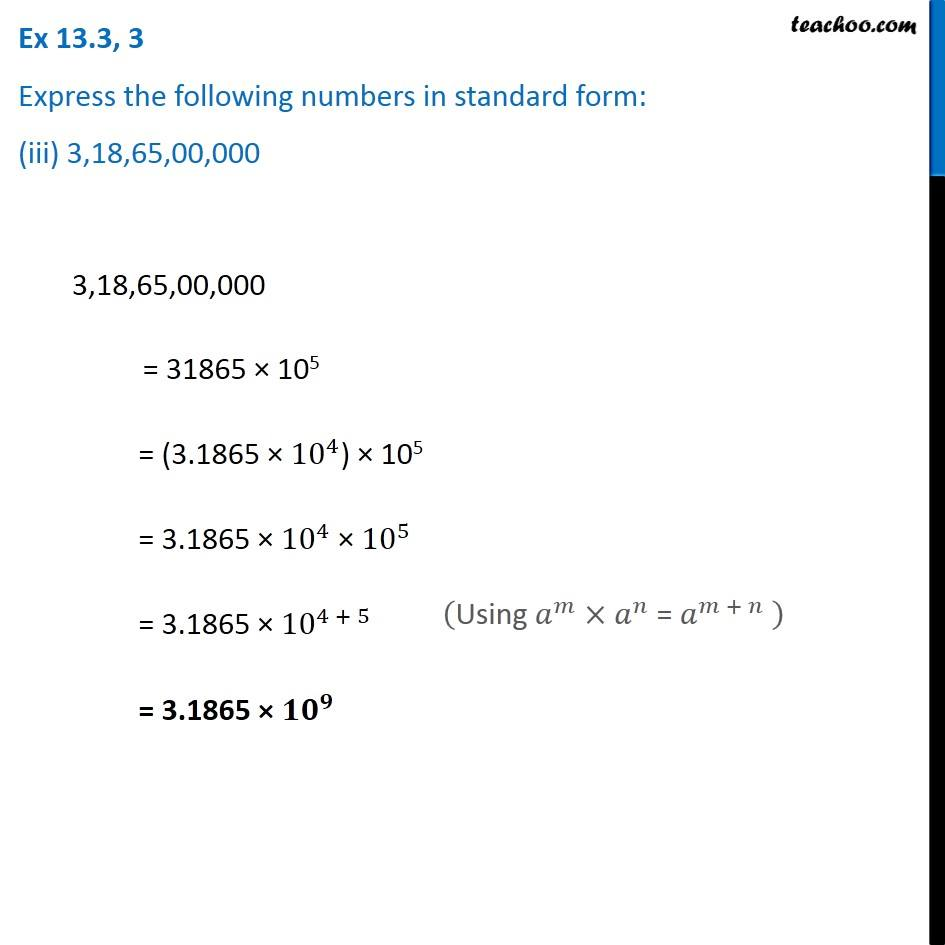Ex 13.3

Chapter 13 Class 7 Exponents and Powers
Serial order wise549 people joined Teachoo Black. What are you waiting for?

### Transcript

Ex 13.3, 3 Express the following numbers in standard form: (iii) 3,18,65,00,000 3,18,65,00,000 = 31865 × 105 = (3.1865 × 〖10〗^4) × 105 = 3.1865 × 〖10〗^4 × 〖10〗^5 = 3.1865 × 〖10〗^(4 + 5) = 3.1865 × 〖𝟏𝟎〗^𝟗 ("Using " 𝑎^𝑚×𝑎^𝑛 " = " 𝑎^(𝑚 + 𝑛) " " )